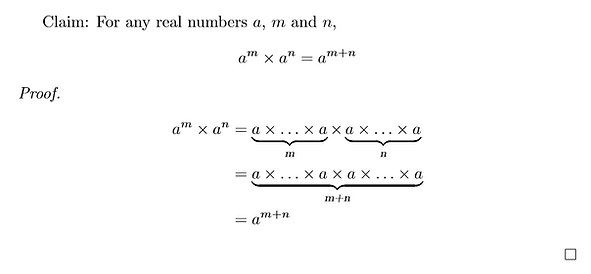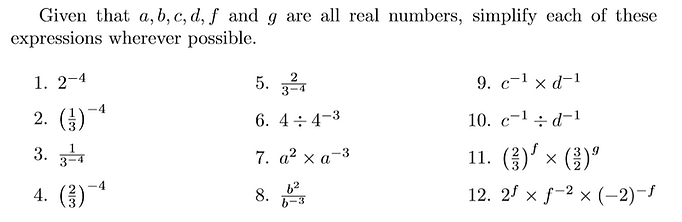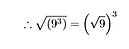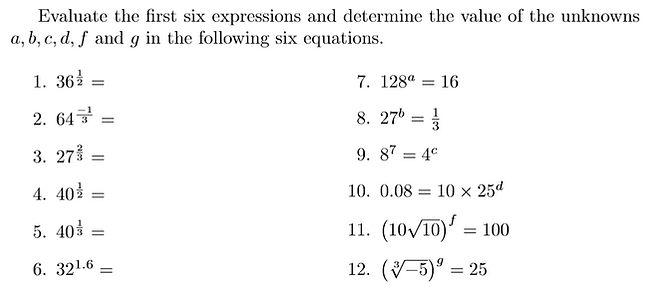top of pagePowers, or indices, are the first mathematical shorthand most of us meet to make the writing of large multiplications of the same term more efficient, they should make our lives easier and yet so many mathematicians get lost with indices. The trick is to remember where they come from; indices and the laws they live by arise from multiplication, a world we know well, so we must always look back there as we seek to understand indices and their behaviour. Here on this page you will explore, practise and prove each of the laws governing indices as they arise and see how each leads to the next. You should also be on the look out for error-tempting moments that have got the better of so many mathematicians before you; if you can explain away their mistakes you will never fall for them yourself.

## The Power is Counting

Once we learn to use indices involving negatives and fractions and so on, there is a temptation always to approach powers from an advanced 'laws of indices' position. In the interest of deep understanding though, it might be wise to start at the very beginning (a very good place to start) so we can be reminded of the meaning of indices and see that their laws are not laws unto themselves but versions of the laws governing all numbers.

Beginning as simply as possible then, what are indices? What do powers do? They count. A power looks at a product and counts the number of appearances of any number or algebraic term, handing us that number of appearances in one neat package so that we need not write them all out. We tend to think of powers in this way when we consider prime factorisation, but in other contexts this thinking can get lost. So often the confusion experienced when interpreting laws of indices can be entirely avoided if we only remember this simple truth; the power is counting the appearances of the term in the product.## The Power of Multiplication

As discussed, raising to a power is about counting in products, so the first thing to investigate should surely be what happens when we take products of products with this shorthand already in play.

Examples of this are quite straight forward to explain, especially if we continue to think of the power as a counter. Before you tackle the general rule, think about how you would explain, for example, why this is true:

With this logic in mind, have a go at proving the general law for multiplying with indices. When you are ready, click the box to reveal the proof.The counter reasoning makes this rule very intuitive:

In the first product the power counts m appearances of a.

In the second product, the power counts n appearances of a.

So in the product of products, the power must count all appearances of a together, m + n appearances of a.

Now that you can prove it, you can use it.## The Power of Division

If there is a rule for multiplication then there must be a rule for division too. This one can seem a little less obvious if we rush too quickly into algebra as the concept of division by cancelling common factors can get lost. Here again then we should look to the explanation of a simple example to inform our thinking about the general rule.

Consider how you would explain each of these equalities:

Now to prove the general rule. Hint: It might help to write in more 'a's than are strictly necessary to help you see what's going on. Click the box to reveal the proof when you're ready.###### 18-16/11

In this proof, writing the power of m as m-n+n might feel a little forced. However, if we return to the idea of the power as a counter, then this reasoning gives us an intuitive sense of what is happening.

When we divide, we cancel common factors. Whenever we cancel the common factor of a, an appearance of a is lost and so the counter is reduced by 1.

Here, we have counted m appearances of a to begin with. We divide by the product where a appears n times, so the common factor of a is cancelled n times, so the counter is reduced by n. We are left with m-n appearances of a.

Thinking in fractions: of the m appearances of a in the numerator, n will be cancelled by the appearances of a in the denominator, so we are left with m-n appearances of a.Now that you can prove it, you can use it.

## The Power of Zero

We can use this division rule to prove the much mis-remembered fact about raising a non-zero number to the power of zero, we have just observed what happens in question 11 above.We can also prove this in lots of similar ways, like this:In this instance, the role of the power as a counter might lead us to expect a different result: if we count 0 appearances of any term in the product then we might think the value of the product should be 0. To understand why this is not the case, we must remember that when we are concerned with multiplication, zero is more of an end than a beginning. If we multiply by zero, we only ever get to zero, regardless of where we start. Therefore, an 'empty' product where no appearances of any term are counted, cannot have value zero; if it did, the product would never have any other value even when appearances of other terms were introduced. An 'empty' product must instead have the 'beginning' value for multiplication, which allows a product to have non-zero value, to have size, but only its own size. The 'empty' product must have value 1. This way, when other terms are introduced to the product, they affect the value as they should.

We can make this same argument algebraically too. For any non-zero term a, raised to any power n:This shows us that when we multiply by a to the power of zero, the value of the product is unchanged.

If a to the power of zero had value zero, then the product would vanish, but we can see that this doesn't happen. Instead, multiplying by a to the power of zero returns the product at its own value. The only real number with this multiplicative property is 1, that is why it is called the 'identity element' of the real numbers under multiplication, multiplying anything by 1 returns the anything as it already was. This also shows us that 1 is where any multiplication must begin if it is going ot go where it should. If we begin at 1 then introducing a takes us to a, then introuding b takes us to ab, then introducing -c takes us to -abc, and so on.

So, the power is still counting appearances of non-zero terms, it's just that when there are none, the beginning value is 1.

This shows us that when we multiply by a to the power of zero, the value of the product is unchanged.

If a to the power of zero had value zero, then the product would vanish, but we can see that this doesn't happen. Instead, multiplying by a to the power of zero returns the product at its own value. The only real number which has this multiplicative property is 1, that is why it is called the 'identity element' of the real numbers under multiplication, multiplying anything by 1 returns the anything as it already was. This also shows us that 1 is where any multiplication must begin if it is going to go where it should. If we begin at 1, then introducing a takes us to a, then introducing b takes us to ab, introducing -c takes us to -abc, and so on.

So, the power is still counting appearances of non-zero terms, it's just that when there are none, the beginning value is 1.

## The Power of Negatives

Another consequence of this division rule, which we observed in Q12 above, is the emergence of negative powers. As ever, negatives here represent taking more than was there to begin with. The power is counting the number of appearances of the term in the product so when the power is negative, we must be counting the number of appearances missing from the product, the number of divisions by the term as opposed to multiplications. As we have observed, each time we divide and cancel a common factor of any term, the power counting appearances of that term is reduced by 1. We have seen that when we divide a non-zero product by itself, the appearances of the term in the numerator and the denominator match, so the power is 0 and the value is 1. Naturally then, if we continue to divide by more appearances of the term, the counter must continue to reduce and so the power becomes negative.

To understand this reasoning, think about how you would explain each of these equalities:

It is likely that you used equivalent fractions or the cancelling of factors to reason the left equality, whereas on the right you simply are bound by the law of dividing with indices which we have proven to be true. In terms of the counter though, the right equality does make sense. If we begin with two 2s in the numerator, these are cancelled by the first two of the seven 2s in the denominator, leaving five 2s in the denominator and a numerator of 1. The counter should therefore show that there are five 2s too few in the product, since we are in fact dividing by those five 2s, hence the power of -5.

Since these two equalities are just different statements of the same truth, we have in fact shown this third equality to be true:

Which leads us to suspect that the general rule should be thus:

To confirm this suspicion we should set about proving that this is the case. We will do so in two rather appealing ways.

Have a go at proving the general rule using the division law of indices.

Hint: It might be useful to remember that - n = 0 - n

Click the box when you are ready to reveal the first proof.

For this second proof we will instead exploit the multiplicative law of indices. Before you begin it might be useful to think back to our previous example. Consider the relationship between these numbers & what that means for their product.

Now you can use exactly the same logic to prove the general rule too. Click the box when you are ready to reveal the second proof.Now that you can prove it, you can use it.## Power to a Power

This next rule is a favourite, a beautifully intuitive instance of what happens when we take a shortcut on a shortcut; double trouble or trouble squared, if you will.

To see what is happening, the simplest tactic is just to write out the meaning of each expression in full.The counter reasoning again makes what happens here very intuitive: A product counting 4 appearances of a product which itself counts 3 appearances of 5 is overall a product counting 12 appearances of 5. A product counting 3 appearances of a product counting 7 appearances of a is a product counting 21 appearances of a. A product counting 2 appearances of a product missing 3 factors of b is a product missing 6 factors of b.

Writing out this process we get the feeling for what is happening. When we raise to a power and then raise that expression to a further power, we end up counting this many lots of that many, so the overall count is the product of the manies.

With this logic in mind, have a go at proving the general law for multiplying with indices. When you are ready, click the box to reveal the proof.This is a deeply useful rule because it gives us a lot of freedom to think about any given power in more than one way. In turn, this freedom can be used as a tool to translate expressions containing indices which appear to have different bases into expressions relating to the same base, unleashing the power (no pun intended) of all our other laws of indices.Now that you can prove it, you can use it.## The Power of Fractions

Now that we know more about positive and negative integer powers, we can use our understanding of these and the rules they've taught us to determine what fractional powers must mean and how they must work. To begin with we'll look at unit fractions (fractions with a numerator of 1) and to investigate these we'll start with a classic half.

We've met a power of a half in Q12 above and even though we don't yet know what a power of a half means, we can find out by investigating what would need to happen to take us from that power of a half to an integer power, since we already understand these. There are lots of options for what we could do to achieve this, but since the power to a power rule gives us the ability to multiply powers, it makes sense to engineer the situation so that the power of a half is multiplied by two, to give the integer power 1. The situation we've engineered is a squaring, just as is given in Q12, so to understand what the power of a half means you should look again at the solution for Q12 and consider inverses to help you work.What this shows us is that 'k to the power of a half' is the thing that squares to give k. We have a catchier name for this; the square root of k. Important sidenote: there are in fact two 'things that square to give k' the positive and negative of the square root of k, but we define k to the power of a half to mean the positive square root because it is necessary and helpful when we have our functions hat on. Linguistically and possible unhelpfully, we talk about *the* square root as if there is only one value with the squaring property, always referring to the positive value, but we must not forget that the negative solution also exists.

We have all the tools now to generalise and form a proof for the meaning of a power of a half. Have a go on your own and then click the box to reveal the proof.If we continue to think of the power as a counter, then when we read a power of a half we learn that we don't have enough to count a whole appearance of the term in the product, but the counter tells us that what we are missing is equal to what we already have. It is therefore clear that multiplying this term by itself (squaring) gives us a whole appearance to count and therefore the power of a half represents a square root. When we're thinking in products, the square root of a factor is 'half way' to a full factor, since two of them takes us there.

It's not too far of a leap now to see what indices of other unit fractions must mean. To help you with your thinking, consider what would happen in each of the below cases and how you might be able to make the same thing happen for a power containing any unit fraction.With this logic in mind, have a go at the proof for a power of a general unit fraction. Click the box when you're ready to reveal the proof.Now that we've covered all unit fractions in indices, we in fact have power over all fractions with any integer in the numerator without having to work much harder. Our access to all other fractions comes courtesy of the power to a power rule which allows us to express any non-unit fraction as an integer multiple of the unit fraction with the same denominator. And, as we should always suspect when multiplication is at play, commutativity strikes again to give us some freedom over how we do so.The power of three halves can therefore be thought of as the square root of the cube or the cube of the square root.Now you're ready for anything that fractional powers can throw at you. Dive in.## The Power to Puzzle

Now that you've met all sorts of rational indices and understand the rules of their behaviour, it would be remiss to move on without taking a moment to play with these new powers of yours and mesure them against some delicious puzzles.Q3: Note that though this may look like a simple 'power to a power' situation, it is in fact subtly different. The second power in each of these expressions is contained within the first. For example, the first one is not 'two to the power of 4, to the power of eight' but rather 'two to the power of, four to the power of eight'. In other words we cannot use 'power to a power' and simplify to two the power of 16, we must work harder than this.

bottom of page